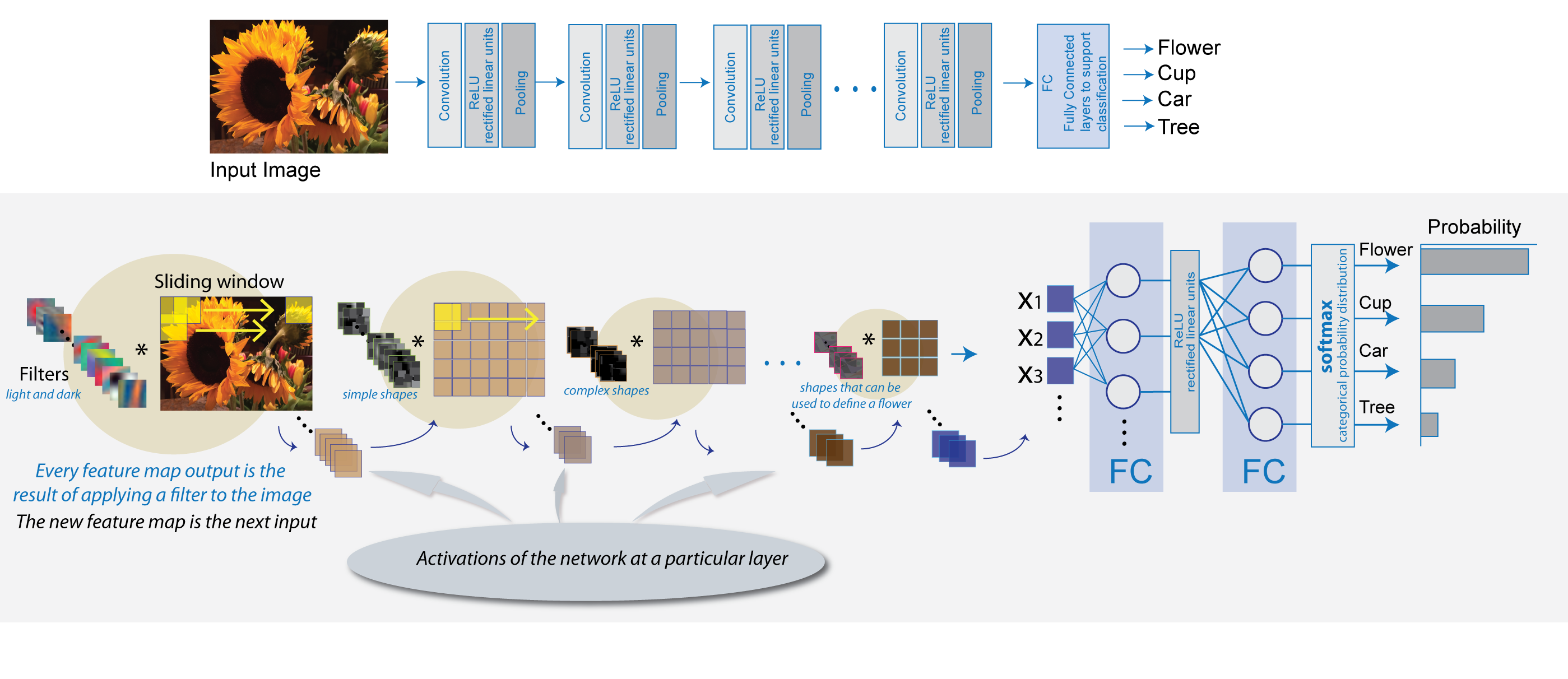## Learn About Convolutional Neural Networks

Convolutional neural networks (ConvNets) are widely used tools for deep learning. They are specifically suitable for images as inputs, although they are also used for other applications such as text, signals, and other continuous responses. They differ from other types of neural networks in a few ways:

Convolutional neural networks are inspired from the biological structure of a visual cortex, which contains arrangements of simple and complex cells . These cells are found to activate based on the subregions of a visual field. These subregions are called receptive fields. Inspired from the findings of this study, the neurons in a convolutional layer connect to the subregions of the layers before that layer instead of being fully-connected as in other types of neural networks. The neurons are unresponsive to the areas outside of these subregions in the image.

These subregions might overlap, hence the neurons of a ConvNet produce spatially-correlated outcomes, whereas in other types of neural networks, the neurons do not share any connections and produce independent outcomes.

In addition, in a neural network with fully-connected neurons, the number of parameters (weights) can increase quickly as the size of the input increases. A convolutional neural network reduces the number of parameters with the reduced number of connections, shared weights, and downsampling.

A ConvNet consists of multiple layers, such as convolutional layers, max-pooling or average-pooling layers, and fully-connected layers.The neurons in each layer of a ConvNet are arranged in a 3-D manner, transforming a 3-D input to a 3-D output. For example, for an image input, the first layer (input layer) holds the images as 3-D inputs, with the dimensions being height, width, and the color channels of the image. The neurons in the first convolutional layer connect to the regions of these images and transform them into a 3-D output. The hidden units (neurons) in each layer learn nonlinear combinations of the original inputs, which is called feature extraction . These learned features, also known as activations, from one layer become the inputs for the next layer. Finally, the learned features become the inputs to the classifier or the regression function at the end of the network.

The architecture of a ConvNet can vary depending on the types and numbers of layers included. The types and number of layers included depends on the particular application or data. For example, if you have categorical responses, you must have a classification function and a classification layer, whereas if your response is continuous, you must have a regression layer at the end of the network. A smaller network with only one or two convolutional layers might be sufficient to learn a small number of gray scale image data. On the other hand, for more complex data with millions of colored images, you might need a more complicated network with multiple convolutional and fully connected layers.

You can concatenate the layers of a convolutional neural network in MATLAB® in the following way:

```layers = [imageInputLayer([28 28 1]) convolution2dLayer(5,20) reluLayer maxPooling2dLayer(2,'Stride',2) fullyConnectedLayer(10) softmaxLayer classificationLayer];```

After defining the layers of your network, you must specify the training options using the `trainingOptions` function. For example,

`options = trainingOptions('sgdm');`

Then, you can train the network with your training data using the `trainNetwork` function. The data, layers, and training options become the inputs to the training function. For example,

`convnet = trainNetwork(data,layers,options);`

For detailed discussion of layers of a ConvNet, see Specify Layers of Convolutional Neural Network. For setting up training parameters, see Set Up Parameters and Train Convolutional Neural Network.

 Hubel, H. D. and Wiesel, T. N. '' Receptive Fields of Single neurones in the Cat’s Striate Cortex.'' Journal of Physiology. Vol 148, pp. 574-591, 1959.

 Murphy, K. P. Machine Learning: A Probabilistic Perspective. Cambridge, Massachusetts: The MIT Press, 2012.